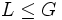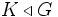# Problems related to subgroup properties

This is a problems page. The solutions can be found at the solutions page

This page lists problems intended to give a better feel of subgroup properties. Before plunging into this, it might be a good idea to have a look at problems in elementary group theory where some very basic questions regarding subgroups are covered. Relevant resources are:

<categorytree>Subgroup properties</categorytree>

The most important of these properties are listed in Category:Pivotal subgroup properties.

## Problems related to normality and related properties

Apart from the main article on normal subgroup, useful resources here are listed in Category:Survey articles related to normality. In particular, refer the article on varying normality

Some very preliminary problems in this area can be seen in Problems in elementary group theory#Normal and characteristic subgroups.

More problems in that direction are listed here.

For most of the problems, the proofs are direct manipulations, but coming out with counterexamples or explicit constructions is not direct, and requires some familiarity with actual groups. When trying to construct counterexamples, start off by looking at Abelian groups (here every subgroup is normal, but not many are characteristic).

### Failure of normality to be transitive

Normality is not transitive: a normal subgroup of a normal subgroup need not be normal. This section explores some problems centered around that:

1. Prove that if$H$ is a direct factor of$G$, and$K$ is a normal subgroup of$H$, then$K$ is normal in$G$. By$H$ being a direct factor, we mean that there exists a subgroup$L \le G$ such that$G$ is an internal direct product of$H$ and$L$
2. More generally, define a subgroup$H$ to be a central factor of$G$ if$HC_G(H) = G$. Prove that$H$ is a central factor of$G$ iff every inner automorphism of$G$ restricts to an inner automorphism of$H$. Further, prove that any normal subgroup of a central factor is normal.
3. Prove that any direct factor, and also any central subgroup (subgroup inside the center), is a central factor.
4. Define a subgroup of a group to be transitively normal if any normal subgroup of it, is normal in the whole group. Prove that the property of being transitively normal is a transitive subgroup property.
5. A subgroup$H$ of a group$G$ is termed conjugacy-closed if whenever two elements of$H$ are conjugate in$G$, they are conjugate in$H$. Prove that any conjugacy-closed normal subgroup is transitively normal.
6. Prove that all the above properties: direct factor, central factor, and conjugacy-closed normal, satisfy the intermediate subgroup condition: if$H$ satisfies the property in$G$,$H$ also satisfies the property in any intermediate subgroup$K$.
7. Suppose$H \le K$ is such that whenever$G$ is a group with$K \triangleleft G$, then$H \triangleleft G$. Then$H$ is a characteristic subgroup of$K$. This is a little harder

### The good things about normality

1. Define a subgroup$H$ of a group$G$ to be potentially characteristic in$G$ if there exists a group$K$ containing$G$ such that$H$ is a characteristic subgroup of$K$. Give an example of a potentially characteristic subgroup that is not characteristic. Show that any potentially characteristic subgroup is normal.
2. Give an example of a group in which the intersection of two direct factors is not a direct factor. Prove that an intersection of direct factors is always a normal subgroup.
3. Give an example of a situation where we have three groups$H \le K \le G$ such that$H$ is normal in$G$,$K$ is characteristic in$G$, but$K/H$ is not characteristic in$G/H$. Prove, however, that$K/H$ is normal in$G/H$.

### Normal subgroups and their permutability properties

1. Give an example of a group$G$ and a subgroup$H$ such that$HK = KH$ for every subgroup$K$, but$H$ is not normal. A subgroup$H$ (normal or not) satisfying the condition$HK = KH$ for all$K$ is termed permutable.
2. Prove that permutability is join-closed: a product of permutable subgroups is permutable. Prove also that permutability satisfies the intermediate subgroup condition: if$H$ is a permutable subgroup of$G$, and$K$ is any intermediate subgroup, then$H$ is permutable inside$K$.
3. A conjugate-permutable subgroup is a subgroup$H$ such that$HH^g = H^gH$ where$H^g$ denotes a conjugate subgroup to$H$. Prove that a product of two conjugate subgroups, each of which is conjugate-permutable, is also a conjugate-permutable subgroup.
4. Prove that a subgroup in a finite group, maximal with respect to the property of being a proper conjugate-permutable subgroup, is normal (you need to use the fact that a product of conjugates is proper).
5. A subgroup is termed 2-subnormal if it is a normal subgroup of a normal subgroup of the whole group. Prove that a subgroup is 2-subnormal iff it is normal inside its normal closure.
6. Prove that any permutable subgroup of a normal subgroup is conjugate-permutable, and hence deduce that any 2-subnormal subgroup is conjugate-permutable.

### Characteristic and normal

One key fact to remember here is that a characteristic subgroup of a normal subgroup is normal.

1. Prove that a group has a nontrivial Abelian normal subgroup if and only if it has a nontrivial nilpotent normal subgroup.
2. Prove that in any group, any minimal normal subgroup is characteristically simple: it has no proper nontrivial characteristic subgroups.
3. Prove that a finite characteristically simple group is a direct product of pairwise isomorphic simple groups (the corresponding result also holds for infinite groups). Hence, show that any nontrivial finite group has a simple 2-subnormal subgroup.
4. Prove that the subgroup generated by two nilpotent normal subgroups is again a nilpotent normal subgroup. Using the quaternion group, show that the corresponding statement does not hold if we replace nilpotent by Abelian.
5. Suppose$G$ can be expressed as the Frattini subgroup of some group. Then show that if$N$ is a characteristic subgroup of$G$,$G/N$ can also be expressed as the Frattini subgroup of some group.